# Permutations

Definition: Combinatorics is a branch of mathematics about the selection and deployment of elements of a set based on some conditions

Selected (or available) group of elements called compounds

## Permutations

Definition: Permutations of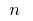the different elements are called finite ordered set (that is, such sets that specify the order of elements) can be removed from a set containingelements (if all elements of a given set of different — get permutations without repetitions, but if in the given set elements may be repeated, then get the permutations with repetitions)

### Formula for the number of permutations#### Without repetitionwhere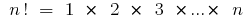(read "n factorial")

For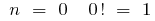(by definition)

Example. The number of different six-digit numbers can be formed from the digitswithout repeating the numbers in one number equal to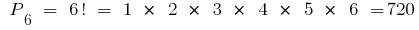#### With repetitionswhereExample.The number of different six-digit numbers can be formed from three twos, two sevens and one five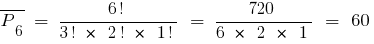(considered)

Tags:
Chapter:
Chapter: Combinatorics
Versions in other languages:
Share with friends: